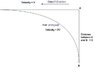# Velocity Problem in Physics

• talksabcd

## Homework Statement

I have a problem in physics that I am struggling to solve.

Consider two objects 1 and 2 positioned at points A and B respectively
on a vertical line. Point A and Point B are separated by a distance X.
object-1 will start moving toward its right at Velocity V and Object-2 starts moving with velocity 2V towards object-1. Here Object-2 from point B will move in such a way that it always travels towards object-1.
So path taken by object-2 will look something like a curve. So Tangent
drawn from object-2 on the curve will always intersect with object-1.

Then

1) At what time both objects will meet ?
2) what will be the equation of the Curve taken by object-2 ?

None

## The Attempt at a Solution

N/A

Ok. Assume that the two objects intersect at a distance x away from the vertical and draw a line joining the starting point of object1 and the piont of intersection. Initially, object 1 was moving in the vertical direction with speed 2V. Take the projection of the velocity along the line you just drew. The total time taken is the length of that line over the projection of the velocity along the line.

I think you got the question wrong. I have attached a diagram which shows the approximate path taken by object2 from point B. Here tangent drawn from object2 will always intersect with object1. Please let me know how to solve the problem.

#### Attachments

•Velocity_Problem.jpg
11.8 KB · Views: 336
Attachment approved. talksabcd, one of the rules here on the PF is that you must show your own work and thoughts in order for us to help you. We do not provide answers -- we can help guide you in your work towards a solution.

So there are Relevant Equations, and you need to show your attempt at a solution. Show us what equations you think apply here...

Hi PF Mentor..

Actually there are no equations/formula for this problem. Finding the equation itself is the problem. I am not even getting the clue on how to approach the problem. The problem looks easy at first glance but when I started solving, nothing is getting materialized.
One thing looks obvious is that I need to use Calculus to solve this problem

Thanks,
talksabcd

Last edited:
Can someone please solve this problem

Can someone please solve this problem

We will not solve it for you. You have to solve it. We're willing to help, but you need to do the work.

One reason that I think not many people have been replying is that your problem statement is confusing. The first few times that I read through it, I thought that it had to have at least one or two incorrect things in it. Now that I've read it another time, I think that maybe the only error is that you say, "object-1 will start moving toward its right at Velocity V", but the diagram shows object-1 moving right to left. Is that the only error?

If so, then start off doing it in discrete steps. Show where O1 is after a short time, and where O2 is at the end of the short time interval (only move them a little bit in each step). Then repeat and see where they end up. Remember the factor of 2x in velocity -- that is what ensures that they will meet.

Show us what your piecewise plot looks like, and what answers you get with it. Then tell us how you think you can convert that into a continuous plot that will get you the exact answers.

What you are describing is a tractrix, also known as a "pursuit curve". They are fun, in a hyperbolic sort of way.

Look in the index of your textbook. That should get you in the right direction. Please come back and post your solution, or at least your attempt at one.

Regards,
Jim